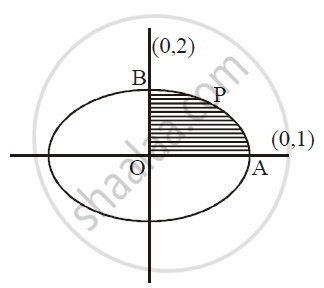# Find the Area of Ellipse X^2/1 + Y^2/4 = 1 - Mathematics and Statistics

Find the area of ellipse x^2/1 + y^2/4 = 1

#### Solution

Required area = 4 Area (OAPB)

= int_0^1 ydx

:. x^2/1 + y^2/4 = 1

:. y =- 2sqrt(1-x^2)

∴Required area = 4int_0^1 2sqrt(1-x^2)dx

= 8[x/2 sqrt(1-x^2) + 1/2 sin^(-1)(x/1)]_0^1

= 8[{0+1/2 sin^1 (1)} - 0]

= 8 xx 1/2.pi/2 = 2pi
sq.units"Concept: Area of the Region Bounded by a Curve and a Line
Is there an error in this question or solution?